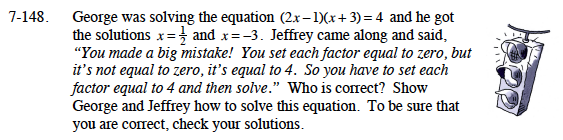Home > A2C > Chapter 8 > Lesson 8.2.3 > Problem8-148

8-148.George was solving the equation (2x − 1)(x + 3) = 4 and he got the solutions x =and x = −3. Jeffrey came along and said, “You made a big mistake! You set each factor equal to zero, but it's not equal to zero, it's equal to 4. So you have to set each factor equal to 4 and then solve.” Who is correct? Show George and Jeffrey how to solve this equation. To be sure that you are correct, check your solutions. Homework Help ✎To use the Zero Product Property, you need a product equal to zero.

Multiply the binomials, subtract 4 to make the equation equal to zero, and factor the resulting trinomial.
Then use the Zero Product Property.